## Designing Freeform Models with 3D Curves

Students: LIU He, YIN Hengli
Advisor: Dr. Chiew-Lan TAI
Computer Science and Engineering Department
Hong Kong University of Science & Technology
[ Introduction ] - [ Modeling Operations ] - [ Differences between Our System and FiberMesh ] - [ Download ] - [ References ]
DIFFERENCES BETWEEN OUR SYSTEM AND FIBERMESH
• Initial Surface Creation
• The FiberMesh’s solution is as follows. First, the system fills the given closed curve with two flat surfaces which have equilateral triangular mesh structures. Then, it uses their non-linear optimization method to inflate the surfaces to obtain a smooth model. (Figure 9)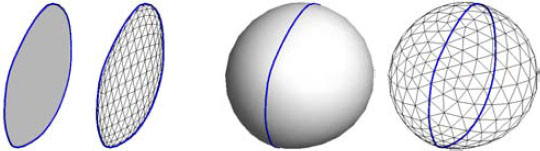Figure 9: Initial Surface Creation in FiberMesh. The flat surfaces with equilateral triangular mesh structures (left) are created and then inflated (right). [Courtesy of Nealen et al]

As stated in the FiberMesh’s paper , their inflation method needs to iteratively solve a few sparse linear systems about 5~10 times to make the surface approach the desired shape.

Our solution is a recursive method. Starting from a closed curve, our system recursively adds a new curve and subdivides the original one into two closed curves until every closed curve contains only three vertices which are used to form a triangle. All triangles combine together to form the mesh structure of the surface. (Figure 10)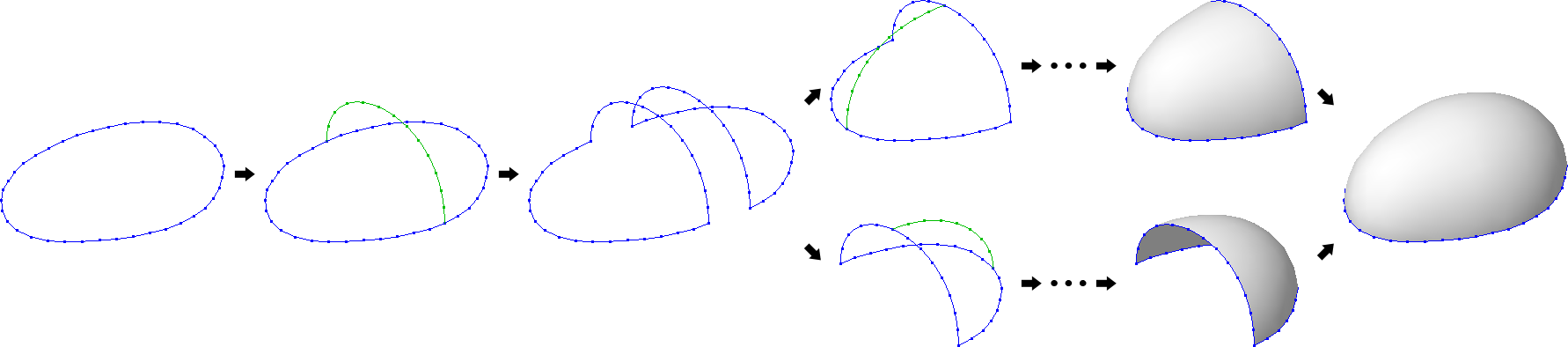Figure 10: Initial Surface Creation in Our System (Single Side). An auxiliary curve (green) is added to the initial closed curve (blue), dividing the closed curve into two. The two new ones are then further recursively divided until each one contains only three vertices, in which case, a triangle is created using the three vertices of each closed curve. Finally, the resulting surface is filled with triangles.

Since the shape of the resulting surfaces depends on the shape of the auxiliary curves added, our system can directly create an inflated initial model. This is much faster than FiberMesh’s iterative inflation method. However, the mesh structure generated by our method is not as regular as FiberMesh. (Figure 11)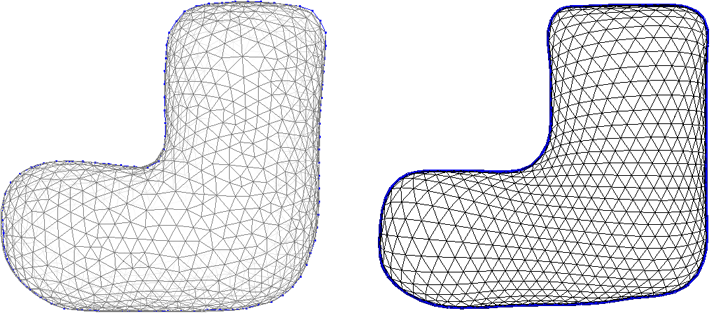Figure 11: Mesh Structure Comparison. The mesh structure generated by our system (left) and the mesh structure generated by FiberMesh (right).

• Surface Smoothing
• A surface smoothing operation is applied after surface creation as well as other editing operations, such as dragging and curve type changing.

FiberMesh’s surface smoothing method is the same as how it inflates the initial model, which is an iterative optimization method. We developed an iterative method to smooth the surfaces.

Our method is an averaging method. The positions of all vertices are updated within each iteration. (Figure 12)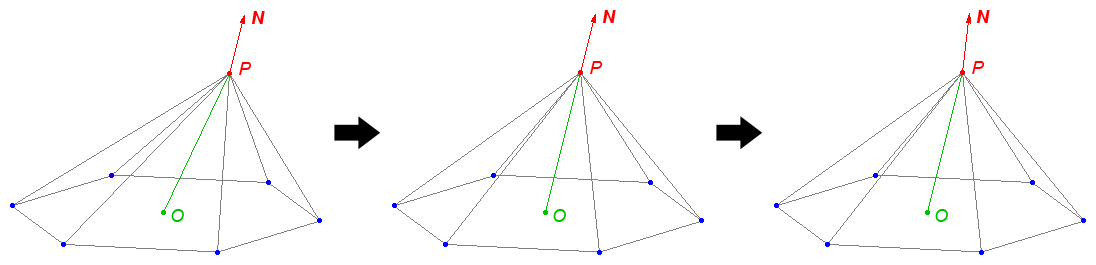Figure 12: Surface Smoothing of a Single Vertex in Our System. The target vertex (P) is moved to a new position, such that OP is parallel with the normal vector N of vertex P, where O is the average position of P’s adjacent vertices (middle). Then, the normal vector is re-calculated for the subsequent iterations (right).

• Control Curve Creation
• FiberMesh does not impose restrictions when the user is drawing a curve. Instead, an undesirable curve is simply removed and a new one redrawn.

We added two functionalities. First, our system ensures that vertices of the curve drawn are equally spaced (Figure 13). Second, our system automatically erases a loop from a self-intersecting curve (Figure 14).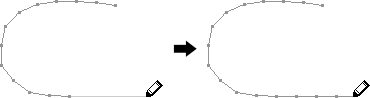Figure 13: When the distance between the cursor and last vertex is more than a threshold, new vertices are added so that adjacent vertices always have equal distance.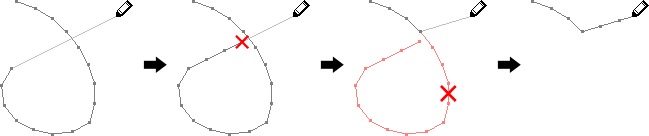Figure 14: Before adding new vertices, the system checks for self-intersection and removes the loop to obtain a simple curve.